# Non Linear Data Structures

## Tree

A tree is a Non-Linear Data Structure that is an abstract model of a hierarchical structure consisting of nodes with a parent-child relation. Its applications are Organization charts, File systems, Programming environments. There are four things associated with any tree -Distinction between nodes, Value of nodes, orientation structure and the number of levels.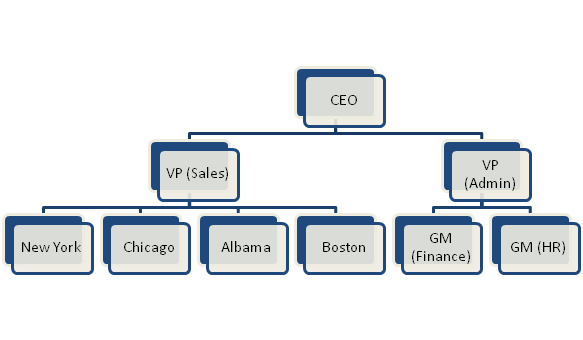### Tree Terminology

• Root– starting point of the tree is a node called root characterized by a node without parent.
• External/leaf node : A node without any children
• Internal node: All the nodes other than the root and leaf nodes. It can be said to be a node with at least one child.
• Ancestors of a node: parent, grandparent and any other nodes which lie on the path from root to that node.
• Depth of a node: number of ancestors for any given node.
• Height of a tree: maximum depth among all the leaf nodes.
• Descendant of a node: child, grandchild and all other nodes lying on path from a node to a leaf node.
• Subtree: tree consisting of a node and its descendants

### Binary Tree

Binary Tree is a tree for which each internal node is allowed to have at most two child nodes. The children of an internal node are called left child and right child depending upon the relationship with the parent. The recursive definition of a binary tree is that it is either a tree consisting of a single node, or a tree whose root has an ordered pair of children, each of which is a binary tree. The applications of Binary Trees are to represent arithmetic expressions, creating decision processes and searching.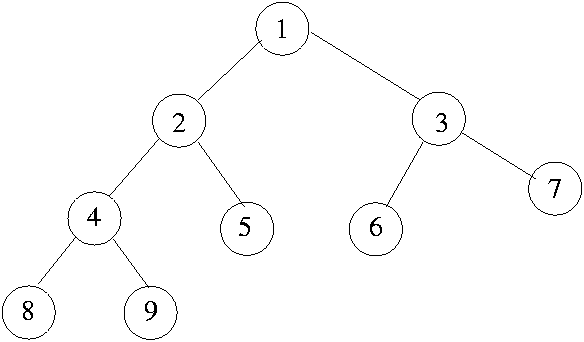• Preorder Traversal– A node is visited before its descendants ( in the example the Preorder traversal sequence is 1,2,4,8,9,5,3,6,7).
• Postorder Traversal– A node is visited after its descendants (in the example the Postorder traversal sequence is 8,9,4,5,2,6,7,3,1).
• Inorder Traversal– A node is visited between traversal of its descendants (in the example the Inorder traversal sequence is 8,4,9,2,5,1,6,3,7).
• If n is the number of nodes, e is the number of external nodes, i is number of internal nodes and h is the height of binary tree then following are the essential properties of binary trees.
• e = i + 1
• n = 2*e – 1
• h>=log2e
• h>=log2(n+1)-1

### Binary Search Tree

It is binary tree with the property that all the nodes to the left of a given node has key values lesser than that node and all nodes to the right have key values greater than the node. An in-order traversal of a binary search tree returns the elements of the BST in ascending order of key values of nodes.Search in a BST for a key k, is done by following a downward path starting at the root. The next node visited depends on the value of the key of the current node. If key of current node is greater than k then the left subtree is searched in same recursive fashion. If key of current node is smaller than k then the right subtree is searched in same recursive fashion. If the key of current node matches the value k then the position of current node is returned. If a leaf node is reached, the key is not found and a null position is returned.

### B-Tree and B+ Tree

A B-tree of order m is an m-way tree (a tree where each node may have up to m children) in which:

• the number of keys in each non-leaf node is one less than the number of its children and these keys partition the keys in the children in the fashion of a search tree
• All leaves are on the same level
• All non-leaf nodes except the root have at least m / 2 children
• The root is either a leaf node, or it has from two to m children
• A leaf node contains no more than m – 1.The number m should always be odd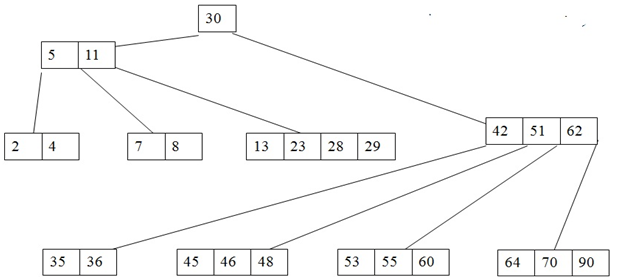• A B+tree is a balanced tree in which every path from the root of the tree to a leaf is of the same length, and each non-leaf node of the tree has between [n/2] and [n] children, where n is fixed for a particular tree. It contains index pages and data pages. The capacity of a leaf has to be greater than or equal to half. Deletion and insertion is very easy to implement.
• On insertion of a value if data page is full but index page is not full then the node must be split and the middle Key should be stored in the sorted index page. Left leaf page contains records with keys below the middle key. Right leaf page contains records with keys equal to or greater than the middle key.
• A B+tree with n=6 the key for each node is between 3 to 6. The index page will be 6+ 1 = 7. Example of a B+ tree with four keys (n = 6) looks like this: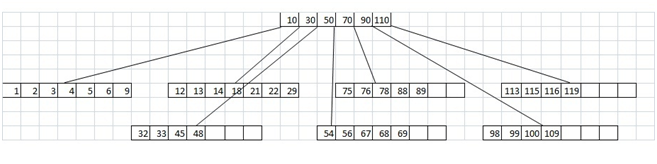## Graph

A graph G(V,E) is a defined with two sets. V , a set of vertices, and E the set of edges between two pair of vertices from the set V.

When the edges of the graph have to be defined with the direction, it is called a directed graph or digraph, and the edges are called directed edges or arcs. In a digraph edge (a,b is not the same as edge (b, a). In a directed edge (a,b) a is called the source/starting point and b is called the sink/destination/ end point. In an undirected graph the direction of edge is not specified.

•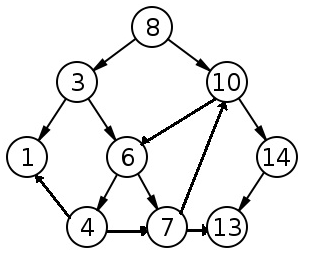When the edges of the graph have to be defined with the direction, it is called a directed graph or digraph, and the edges are called directed edges or arcs. In a digraph edge (a,b is not the same as edge (b, a). In a directed edge (a,b) a is called the source/starting point and b is called the sink/destination/ end point. In an undirected graph the direction of edge is not specified.Two vertices that are connected t each other with an edge are called neighbors.They are also said to be adjacent to each other.

## One Comment

1.Alexis Schultheiss said:

An exceptionally good post. This information sums up for me what this topic depends upon and some of the essential benefits that can be created from knowing about it as you. A friend once remarked that you have a totally different perspective when you do something for certain as opposed to when you’re just toying with it. In the case of this topic, I believe you are taking, or start to consider, a more professional in addition to thorough approach to either what and how you write, which in turn helps you to carry on and get better and help others who don’t know everything about what you have discussed here. Thank you.

October 31, 2019